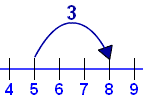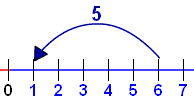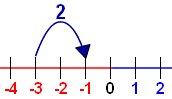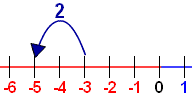# Using The Number Line

images/number-line.js?mode=int

We can use the Number Line to help us add:### 5 + 3 = 8

And subtract:### 6 − 5 = 1

It is also great to help us with negative numbers:### −3 − 2 = −5

So when we get puzzling questions like "What is −6 plus 3" we can use the number line!

## More Examples:

images/number-line-using.js?mode=ex

## Try It Yourself:

Drag the points, see how it all works:

images/number-line-using.js?mode=user

## What Is Next

Sometimes we get questions like

3 + (−6)

Plus and Minus together?

Read How to Add and Subtract Positive and Negative Numbers to find what to do.

1977, 1978, 1979, 1980, 5062, 5063, 5064, 5065, 5066, 5067